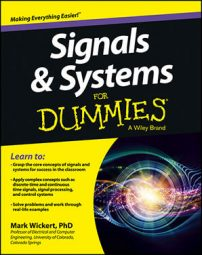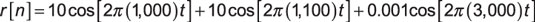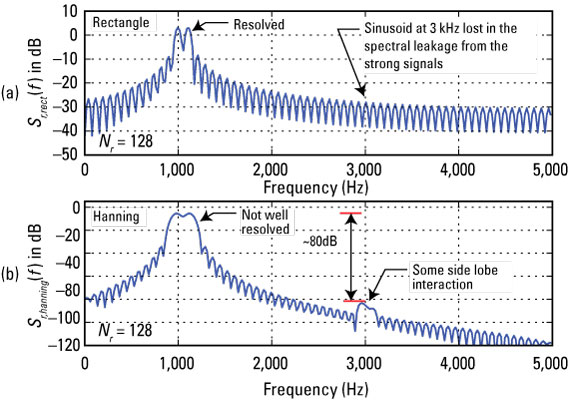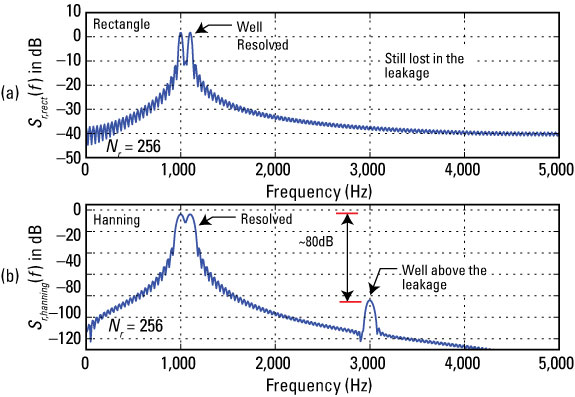##### Signals and Systems For DummiesTo finish off this case study, simulate the system in Python. To give you a feel for sinusoidal spectrum analysis and window selection, here’s a Python simulation that utilizes the test signal:Assume that the sampling rate is 10 kHz, which is greater than twice the highest frequency of 3,000 Hz. The first challenge is resolving the two equal amplitude sinusoids at 1,000 and 1,100 Hz (f = 100 Hz). The second challenge is the 3,000-kHz sinusoid that’s down 80 dB in amplitude relative to the other two.

Use the custom Python function f,Sx = ssd.simple_SA(x,NS,NFFT,fs,window='boxcar') to compute the results. The window functions come from scipy.signal and the FFT (efficient version of required DFT) from the NumPy fft library: X = fft.fft(w*x,NFFT).

Start with Nr = 128 and zero pad (appending 512 – Nr zeros samples) the FFT length to 512 to allow greater spectral interpolation. If more data is available, use it.

```In [<b>870</b>]: f,Sr = ssd.simple_SA(r,128,512,10000,
window='boxcar') # second ‘hanning’```

The following figure shows the estimated spectrum for the rectangle and Hanning windows.Credit: Illustration by Mark Wickert, PhD

Figure (a) shows that the 1,000- and 1,100-Hz sinusoids are resolved; this is not the case in Figure (b) because of the difference in the main lobe width.

Out at 3,000 Hz, dynamic range issues are caused by spectral leakage. Leakage is spectral content that spreads the side of the sinusoid frequency; it’s a function of the window shape and the window length. The spectral leakage from the large amplitude sinusoids at 1,000 and 1,000 Hz is spreading out to 3,000 Hz; leakage covers the spectrum of the 3,000-Hz sinusoid.

The side lobes of the rectangular window fall off at only 6 dB/octave; they fall off at 18 dB per decade in the Hanning window. This explains why in Figure 3b the 3,000-Hz sinusoid is poking through the leakage from the strong signals. Windows give you options.

Repeat the experiment with Nr = 256 and check your results against this figure.Credit: Illustration by Mark Wickert, PhD

Doubling the window length improves the spectral resolvability of the closely spaced sinusoids. The dynamic range is also improved with the larger window length, making the weak sinusoid stand up above the spectral leakage.